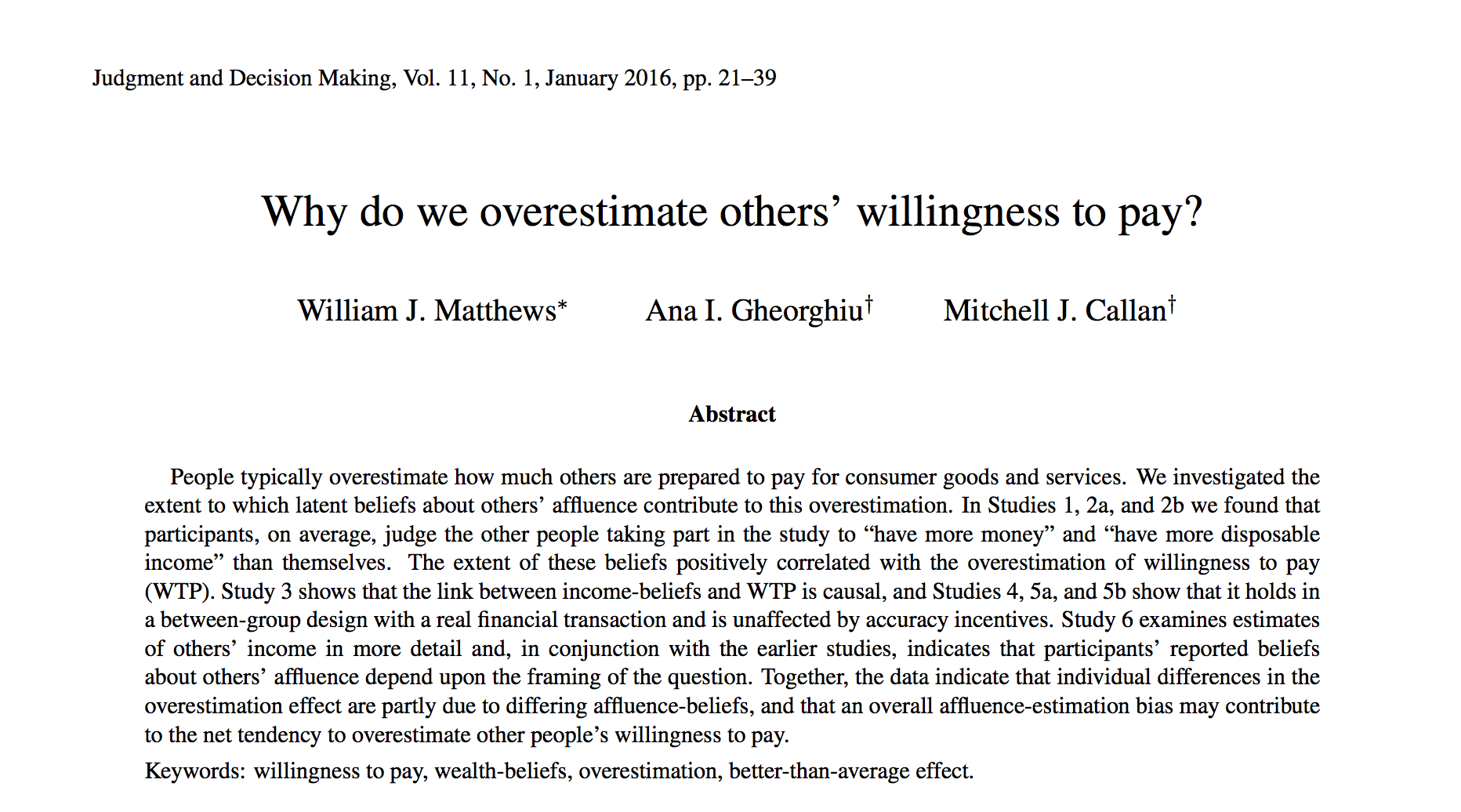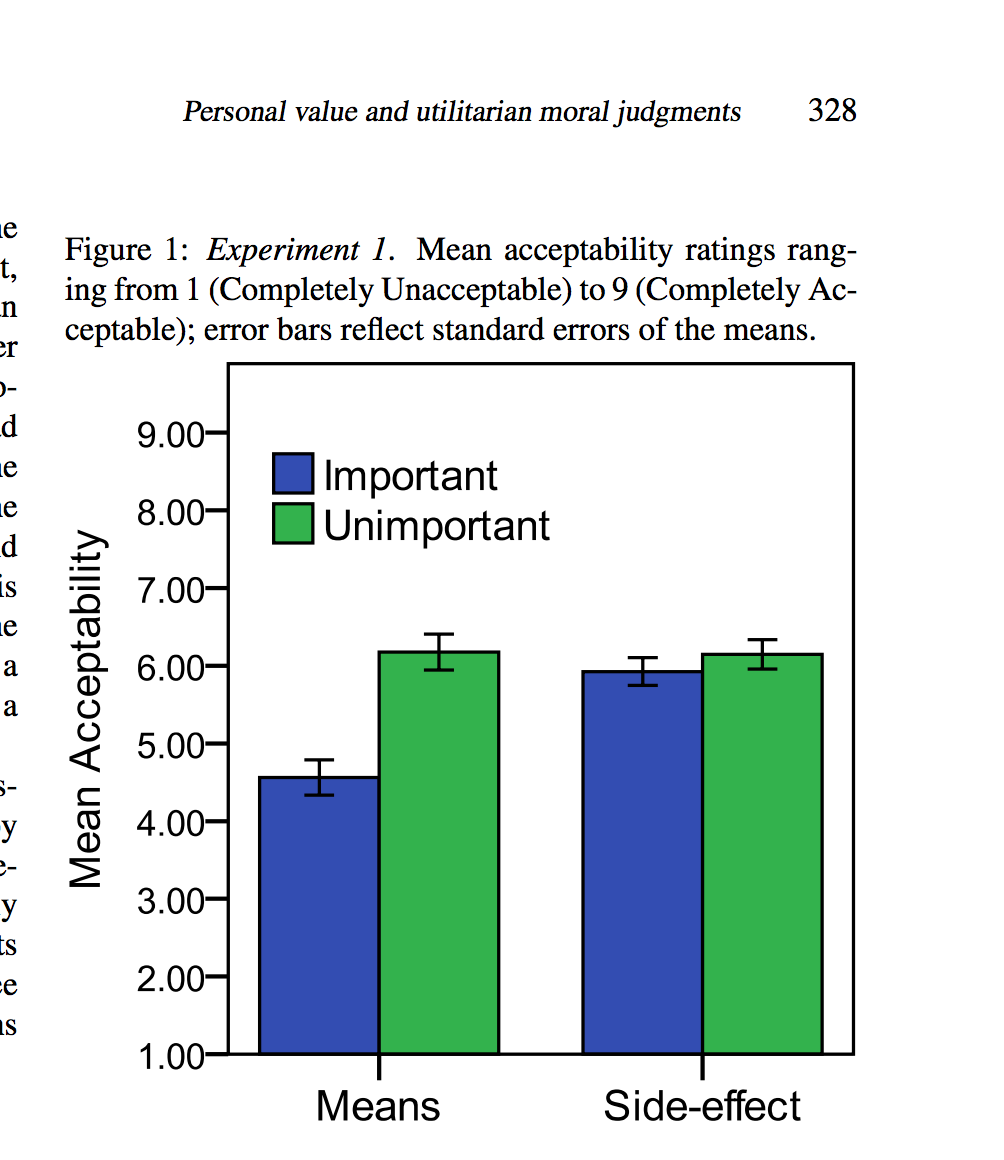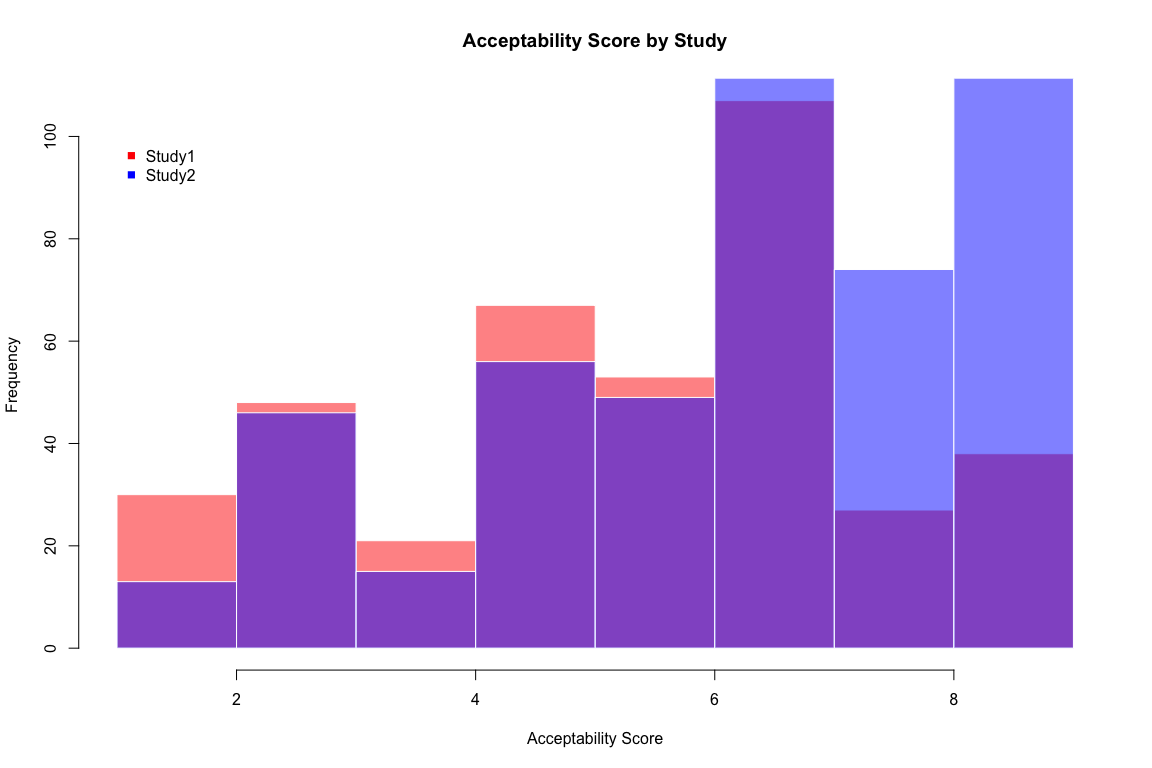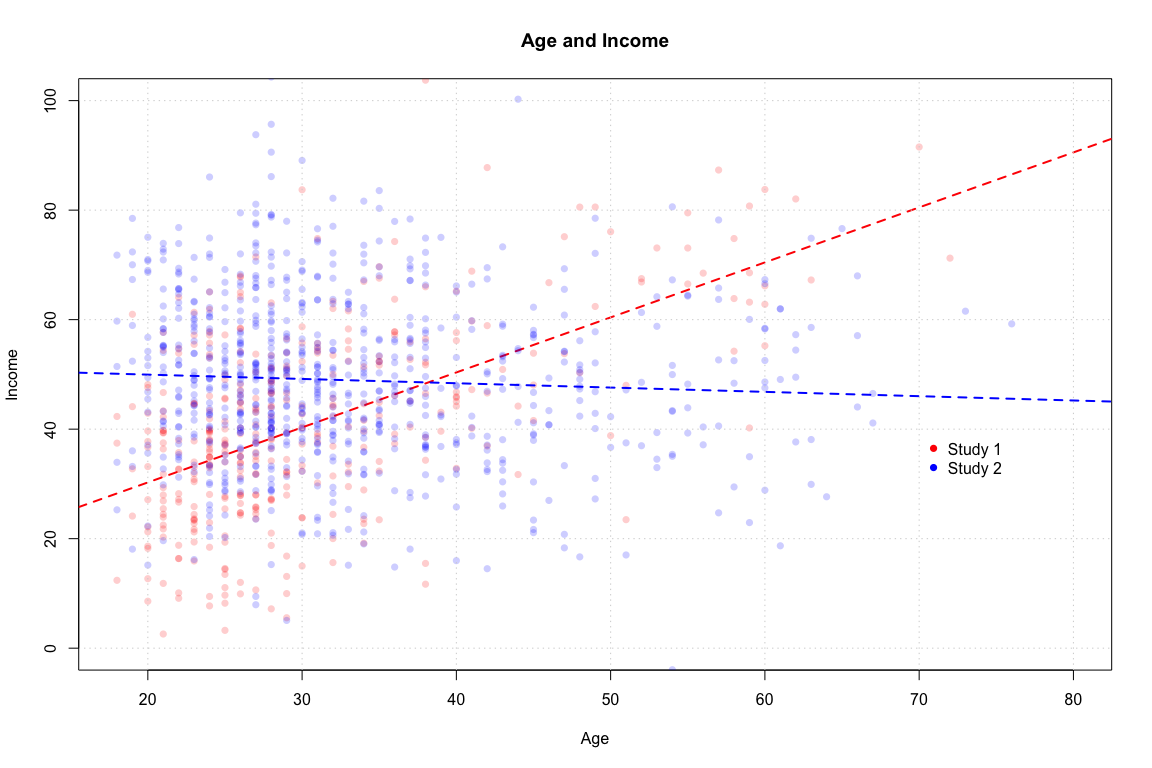## It’s personal: : The effect of personal value on utilitarian moral judgmentsIn this WPA, we will analyze data from Millar et al. (2016): It’s personal: : The effect of personal value on utilitarian moral judgments.

Here is the abstract (You can find the full paper at http://journal.sjdm.org/16/16428/jdm16428.pdf):

We investigated whether the personal importance of objects influences utilitarian decision-making in which damaging property is necessary to produce an overall positive outcome. In Experiment 1, participants judged saving five objects by destroying a sixth object to be less acceptable when the action required destroying the sixth object directly (rather than as a side-effect) and the objects were personally important (rather than unimportant). In Experiment 2, we demonstrated that utilitarian judgments were not influenced by the objects’ monetary worth. Together these findings suggest that personal importance underlies people’s sensitivity to damaging property as a means for utilitarian gains.

# Data

Variable Description
Acceptability.Score How acceptable is the action?
Important Were the objects important to the owner or not?
Direct Was the destruction of an object a means of saving the others or a side-effect?
Cover.Story Was the object a poster or a clock?
Gender Participant gender
Age Participant Age
TopicCompQ Comprehension question 1
ExpensiveCompQ Comprehension question 2
ImportanceCompQ Comprehension question 3
Failed.controls. Did participant fail an attention check?
Variable Description
AcceptabilityScore How acceptable is the action?
Important Were the objects important to the owner or not?
Direct Was the destruction of an object a means of saving the others or a side-effect?
Expensive Was the object expensive or not?
PreviousTrolley Did participants complete a trolley problem in the past?
Gender Participant gender
Age Participant Age
TopicCompQ Comprehension question 1
ExpensiveCompQ Comprehension question 2
ImportanceCompQ Comprehension question 3
Failed.controls. Did participant fail an attention check?
1. You’ll need the latest version of the yarrr package (v0.1.2) in this WPA. Install the package from CRAN with install.packages() then load the package with library()!

2. Open your R project from last week (I recommended calling it RCourse or something similar). There should be at least two folders in this working directory: data and R.

3. Open a new R script and save it as wpa4.R in the R folder in your project directory

4. The data are stored in two separate .csv files. Study 1 is at http://journal.sjdm.org/16/16428/expt1.csv and Study 2 is at http://journal.sjdm.org/16/16428/expt2.csv. Load the data into R by using read.table() (or the Import Dataset button in RStudio) into new objects called study1.df and study2.df. Note: You can either read the tables directly from the web, or download the files to your computer, and then load them (Hint: Make sure to use the arguments sep = "," and header = TRUE)

5. Look at the structure of each data frame using str(). Do you notice something strange about the class of the Acceptability Score column? Run a frequency table of the data with table() to see what’s going on.

6. The data frames have a few miscoded values. To see this, look at the last few rows of the files as follows:

# Look at the last few rows of both dataframes
tail(study1)
tail(study2)
1. As you can see, there are some notes at the bottom of the files. We’ll have to fix this by manually going through each column and setting invalid values to NA. To do this, we’ll create a custom recoding function called recode.v that takes a vector as an argument, and returns a vector with old values recoded to new ones. Run the following code to create our new recoding function recode.v
# JUST COPY, PASTE, AND RUN!

# Create a function called recode.v

recode.v <- function(x,    # What vector do you want to recode?
old,  # What values do you want to change?
new,  # What should the new values be?
otherNA = TRUE,  # Should other values be converted to NA?
numeric = TRUE) { # Should result be numeric?

# Copy vector to x.new
x.new <- x

# Remove factors
if(class(x.new) == "factor") {x.new <- paste(x.new)}

# Loop over all old values
for(i in 1:length(old)) {

x.new[x == old[i]] <- new[i]

}

# Convert unspecified values to NA?
if(otherNA) {

x.new[(x %in% old) == FALSE] <- NA

}

# Convert vector to numeric?
if(numeric) {x.new <- as.numeric(x.new)}

# Return new vector!
return(x.new)

}
1. Here’s how recode.v works. Run the following code and look at the output
# JUST COPY, PASTE, AND RUN!

# recode a string vector of gender data to 0, 1
recode.v(x = c("m", "male", "f", "f", "female", "m", "other", "g;"),
old = c("m", "male", "f", "female"),
new = c(0, 0, 1, 1))
1. Now that you’ve created recode.v, let’s apply it to all the data columns! Run the following code to clean the data.
# JUST COPY, PASTE, AND RUN!

## STUDY 1 CLEANING
study1$Acceptability.Score <- recode.v(study1$Acceptability.Score, old = 1:9, new = 1:9)
study1$Important <- recode.v(study1$Important, old = c("1", "1-yes", "2", "2-no"), new = c(1, 1, 2, 2))
study1$Direct <- recode.v(study1$Direct, old = c("1", "1-yes", "2", "2-no"), new = c(1, 1, 2, 2))
study1$Cover.Story <- recode.v(study1$Cover.Story, old = c("1", "1-poster", "2", "2-clock"), new = c(1, 1, 2, 2))
study1$Gender <- recode.v(study1$Gender, old = c("1", "1-male", "2", "2-female"), new = c(1, 1, 2, 2))

## STUDY 2 CLEANING
names(study2) <- "Acceptability.Score"   # The name for study2 was not the same as study1!
study2$Acceptability.Score <- recode.v(study2$Acceptability.Score, old = 1:9, new = 1:9)
study2$Important <- recode.v(study2$Important, old = c("1", "1-yes", "2", "2-no"), new = c(1, 1, 2, 2))
study2$Direct <- recode.v(study2$Direct, old = c("1", "1-yes", "2", "2-no"), new = c(1, 1, 2, 2))
study2$Expensive <- recode.v(study2$Expensive, old = c("1", "1-yes", "2", "2-no"), new = c(1, 1, 2, 2))
study2$PreviousTrolley <- recode.v(study2$PreviousTrolley, old = c("1", "1-yes", "2", "2-no"), new = c(1, 1, 2, 2))
study2$Gender <- recode.v(study2$Gender, old = c("1", "1-male", "2", "2-female"), new = c(1, 1, 2, 2))
study2$TopicCompQ <- recode.v(study2$TopicCompQ, old = c("2", "4", "4-dolly/brakes"), new = c(2, 4, 4))
study2$ExpensiveCompQ <- recode.v(study2$ExpensiveCompQ, old = c("1", "1-expensive", "2", "2-inexpensive"), new = c(1, 1, 2, 2))
study2$ImportanceCompQ <- recode.v(study2$ExpensiveCompQ, c("1", "1-yes", "2", "2-no"), new = c(1, 1, 2, 2))
1. Now look at the structure of the dataframes with str(), and the last few rows with tail(). Do they look ok now?

## Histograms

1. Create a histogram of the acceptability scores from study 1. Add appropriate labels and colors as you see fit!
# FIX THE CODE BY REPLACING ZZZ WITH THE CORRECT ARGUMENTS

hist(x = ZZZ
main = "ZZZ",
xlab = "ZZZ",
col = "ZZZ")
1. Now do the same for study 2. And for this plot, add a vertical line at the mean of the distribution with abline() (or segments())
# FIX THE CODE BY REPLACING ZZZ WITH THE CORRECT ARGUMENTS

hist(x = ZZZ
main = "ZZZ",
xlab = "ZZZ",
col = "ZZZ")

# Add vertical line at mean of the distribution
abline(v = ZZZ,
lty = 2)

## Scatterplots

1. Create a scatterplot showing the relationship between age and acceptability score in study 1. Add appropriate labels!
# FIX THE CODE BY REPLACING ZZZ WITH THE CORRECT ARGUMENTS

plot(x = ZZZ,
y = ZZZ,
xlab = "ZZZ",
ylab = "ZZZ",
main = "ZZZ")
1. Now, make the plot look a bit nicer! Try changing the point types (e.g.; pch = 16), point colors (e.g.; col = gray(.0, .2), or col = transparent("blue", .5))

2. Now add grid lines with grid() (Hint: Just evaluate grid() after your plot!)

3. Now let’s add a regression line to the plot. Adding a regression line is easy. First, create a linear model object created with lm(). Then add the model to the plot with abline():

# JUST COPY, PASTE, AND RUN!

# Create a regression model
model <- lm(Acceptability.Score ~ Age,
data = study1)

# Add the model to the plot!
abline(model,
lwd = 2,
col = "red")
1. Now create the same scatterplot for the study 2 data. Be sure to include the gridlines and regression line

## Barplot

The authors present the following barplot on page 328, let’s try to represent the same data with a barplot in R:1. In order to create a stacked barplot with the barplot() function, we first need to create a matrix of values. Run the following code to calculate a matrix of mean acceptability scores as a function of Important and Direct with aggregate() and cbind():
# JUST COPY, PASTE, AND RUN!

# Create a matrix of group means
s1.as.means <- aggregate(Acceptability.Score ~ Important + Direct,
FUN = mean,
data = study1)

s1.as.means.mtx <- cbind(s1.as.means[1:2, 3], s1.as.means[3:4, 3])
colnames(s1.as.means.mtx) <- c("Means", "Side Effect")
rownames(s1.as.means.mtx) <- c("Important", "Unimportant")
1. Now create a barplot from the study 1 data by entering the correct arguments in the following code
# FIX THE CODE BY REPLACING ZZZ WITH THE CORRECT ARGUMENTS

barplot(height = s1.as.means,
beside = TRUE,
legend.text = TRUE,
ylim = c(ZZZ, ZZZ),
ylab = "ZZZ")
1. Now, repeat the process to get the same plot from study 2:

## pirateplot

1. Now create a pirateplot of the same data from study 1 (see the help menu by running ?pirateplot). Add appropriate labels and feel free to use your favorite theme (1, 2, or 3), and palette from the piratepal() function like "evildead" or "pony"! (run piratepal("all") to see all of the palettes!
pirateplot(formula = ZZZ ~ ZZZ,
data = ZZZ,
pal = ZZZ,
theme = ZZZ)
1. Now do the same from study 2. Again, add appropriate labels and feel free to use your favorite palette or theme.

# CHECKPOINT!

If you got this far you’re doing great!

## 2 variable histogram

1. Create the following overlapping histogram showing the distribution of acceptability scores between the two studies:
# FIX THE CODE BY REPLACING ZZZ WITH THE CORRECT ARGUMENTS

# study1 acceptability scores
hist(x = ZZZ,
col = transparent("blue", .5),
border = "white",
xlab = "ZZZ",
main = "ZZZ")

# study2 acceptability scores
hist(x = ZZZ,
col = transparent("red", .5),
border = "white",

legend(x = 1,
y = 100,
legend = c("ZZZ", "ZZZ"),
col = c("blue", "red"),
pch = c(15, 15),
bty = "n")## Scatterplot with reference lines

1. Add a new column to study2$income that shows income as a function of Age as follows: # JUST COPY, PASTE, AND RUN! # Add a new column to study 1 called Income study1$Income <- study1$Age + rnorm(n = nrow(study1), mean = 10, sd = 15) # Add a new column to study 1 called Income study2$Income <- 40 + rnorm(n = nrow(study2), mean = 10, sd = 15)
1. Now, create the following scatterplot using the following template:# FIX THE CODE BY REPLACING ZZZ WITH THE CORRECT ARGUMENTS

#  Create a blank plot with labels
plot(x = 1,
y = 1,
xlim = c(ZZZ, ZZZ),
ylim = c(ZZZ, ZZZ),
xlab = "ZZZ",
ylab = "ZZZ",
main = "ZZZ",
type = "n")

points(x = ZZZ,
y = ZZZ,
pch = 16,
col = transparent("red", .8))

# Add regression line for study 1
abline(lm(Income ~ Age, data = study1),
col = "red", lty = 2, lwd = 2)

points(x = ZZZ,
Y = zzz,
pch = 16,
col = transparent("blue", .8))

# Add regression line for study2
abline(lm(Income ~ Age, data = study2),
col = "blue", lty = 2, lwd = 2)

legend(x = 70,
y = 40,
legend = c("ZZZ", "ZZZ"),
pch = 16,
col = c("blue", "red"),
bty = "n")

grid()

## Balloon plots

1. You can have a lot of fun with plots. Let’s make a new type of plot called a balloon plot. A balloon plot will be a customized scatterplot where points are shown as balloons. In the code below, I’ll create a new custom function (we’ll get to this later in the course) called balloonplot(). Look at the code to see how it generally works. Then, copy, paste, and run the code in your script.
# JUST COPY, PASTE, AND RUN!

# Create balloonplot(), a wrapper function for plot() that turns points into balloons!

balloonplot <- function(
x, y,    # x and y vectors
cex = 1, # point sizes
col = piratepal("basel"), # color
... # Other arguments passed to plot()
) {

# Set up the plotting space
plot(1,
bty = "n",
xlim = c(min(x), max(x)),
ylim = c(min(y), max(y)),
type = "n",
...
)

segments(x0 = x + rnorm(length(x), mean = 0, sd = .3),
y0 = y - cex * diff(range(y)) / 25,
x1 = x,
y1 = y,
col = gray(.5, .5),
lwd = cex / 4
)

points(x,
y,
cex = cex, # Size of the balloons
pch = 21,
col = "white", # white border
bg = col)

grid()
}
1. Now let’s try our new balloonplot() function on some data! Run the following to create a balloon plot of the relationship between pirate’s height and weight:
# JUST COPY, PASTE, AND RUN!

balloonplot(x = pirates$height, y = pirates$weight,
cex = 1.5,
col = piratepal("xmen", .3),
xlab = "Height",
ylab = "Weight",
main = "Balloon plot of pirate data")
1. Now, create a balloon plot of the age and income data from study 1! Play around with col and the cex arguments to see how the plot changes.

## You pick the plot

For the following exercises, make a plot that you think represents the data bast.

The movies dataframe in the yarrr package contains data about the top 5000 grossing movies of all time. You can learn more about the data using the help menu ?movies

1. Create a plot that shows the relationship between a movie’s release year and its running time. Customise it and make it look nice!

2. Create a plot that shows the relationship between a movie’s budget and its revenue. Customise it and make it look nice!

3. Create a plot that shows the relationship between genre and time. Customise it and make it look nice! (Hint: You may notice that many of the times are equal to 0, try creating the plot after excluding these values using subset.)

## Submit!

Save and email your wpa_X_LastFirst.R file to me at nathaniel.phillips@unibas.ch. Then, go to https://goo.gl/forms/UblvQ6dvA76veEWu1 to complete the WPA submission form.